# Two-Dimensional Space Groups

Steven Dutch, Professor Emeritus, Natural and Applied Sciences, University of Wisconsin - Green Bay

## What Kinds of Two-Dimensional Symmetry are Possible?

If we are only concerned about a single point, any symmetry is possible in two dimensions; a flower can have any number of petals. But if the pattern repeats, then the possibilities are very limited. Consider the diagram below:The red point is an axis of n-fold symmetry, and so is every other point. (The pattern repeats, after all.) So the first point gets rotated to the second blue point (among others), and the first green point gets rotated to the second green point.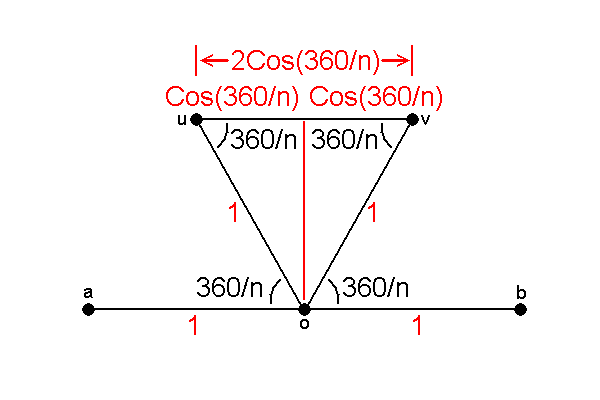Now in this repeating pattern, all the points are spaced the same distance apart in the rows. Call that distance 1. Points u and v must be an integer distance apart. In the sketch they are distance 1 apart but in a square lattice, for example, they might coincide. Thus distance uv = an integer = 2 cos (360/n)

Now the cosine can only have values between -1 and 1, so 2 cos a, which must be an integer, can only take on values -2, -1, 0, 1 and 2. Thus cos a can only be -1, -1/2, 0, 1/2 and 1, or a = 180, 120, 90, 60 and 0 (or 360), respectively. Since the rotations are symmetry rotations,
a = 360/n, so n = 2, 3, 4, 6, or 1

Thus, repeating patterns in the plane can only have 1, 2, 3, 4 or 6-fold symmetry. In particular, repeating patterns in the plane cannot have five-fold symmetry.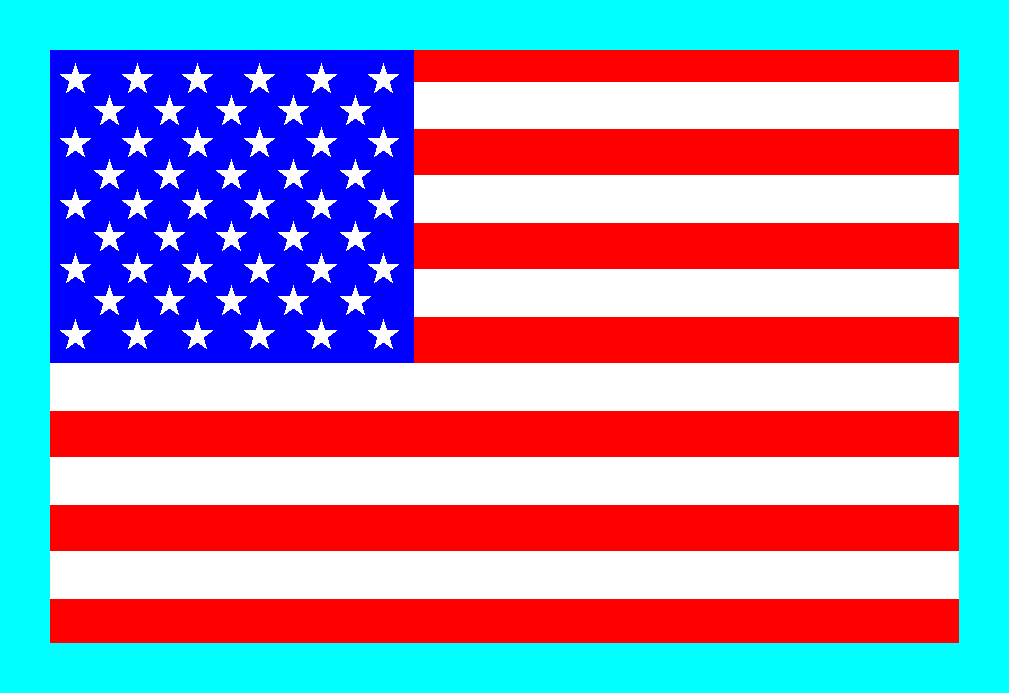• What about the stars in the flag? The stars have five-fold symmetry, but the overall symmetry of the pattern does not, as seen above. Note that rotating the flag 72 degrees preserves the appearances of the stars, but not their overall pattern
• In recent years some fascinating materials have turned up with five-fold symmetry. These materials, termed quasicrystals, do not have repeating patterns. No natural materials have been found with quasicrystal symmetry

## What Combinations of Two-Dimensional Symmetry are possible?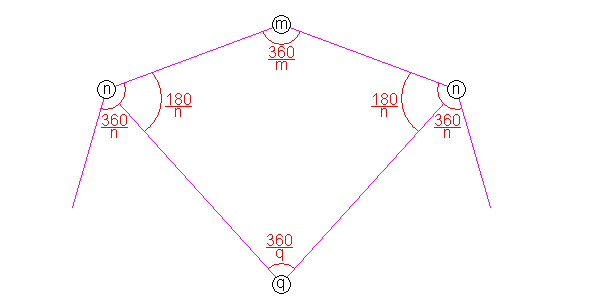Above, we have an n-fold and m-fold rotation axis acting on one another. n and m may or may not be equal. The n-fold axis rotates the m-fold axis to a new location, which then in turn rotates the n-fold axis, and so on. Eventually the circuit will close, and at the center of the circuit will be a symmetry axis. Call this symmetry value q. Now the angles in the quadrilateral sum to 360/n + 360/m + 360/q = 360, or 1/n + 1/m + 1/q = 1.

Trying the permissible crystallographic symmetries for n and m, we find:

• 2,3,6
• 2,4,4
• 3,3,3

## The five basic lattice types

There are 17 space groups in the plane, but their unit cells fall into one of five basic shapes as follows:• General Parallelogram lattice (Green)
• General Rectangular Lattice (magenta)
• General Rhombic (Centered) Lattice (yellow) The simplest unit cell for this pattern is a rhombus, but the pattern also has a rectangular structure. To bring out the rectangular pattern, this lattice is often described as a rectangle with an extra point in the center. Such a lattice is called centered.
• Square Lattice (blue) The lattice of a checkerboard or graph paper.
• Hexagonal Lattice (red) Note that there are three equivalent ways to orient the unit cells. This is the lattice for honeycombs.

## The 17 Plane Space Groups

### Parallelogram and Rectangular Lattices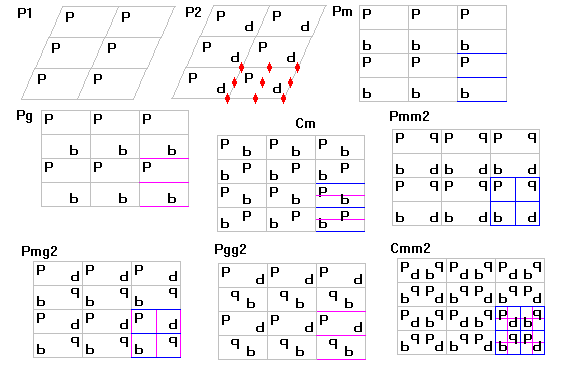#### Simple Parallelogram Lattices

P1 has no symmetry. Note, if the parallelogram happens to be a rectangle or even a square, but the motif has no symmetry, the pattern is still P1. P2 has 2-fold axes.

#### Rectangular Lattices

pm has Parallel mirror planes

pg has Parallel glide planes

pmm has Perpendicular mirror planes

pmg has Perpendicular mirror and glide planes

pgg has Perpendicular glide planes. (p,b) and (d,q) are related by horizontal glides, (p,q) and (b,d) by vertical glides. Note that the intersections of the glide planes and the centers of the boxes outlined by the glide planes are also two-fold symmetry axes.

### Centered Lattices

cm has Parallel alternating mirror and glide planes. The pattern of stars on a 50-star flag has this symmetry.

cmm has Alternating mirror and glide planes in both directions. All intersections are also two-fold symmetry axes. Bricks in a wall have this symmetry.

The figure below gives examples of each pattern.### Square LatticesExamples of each symmetry are shown below.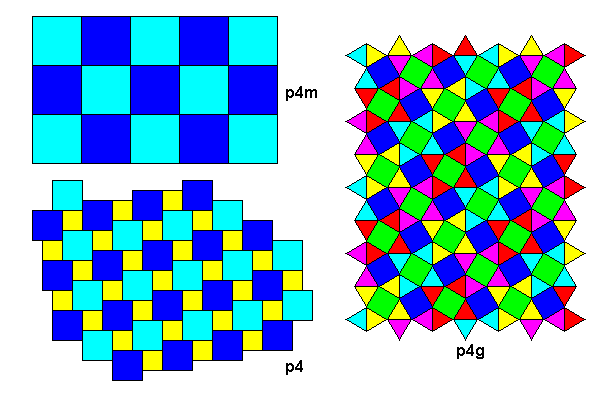### Trigonal Lattices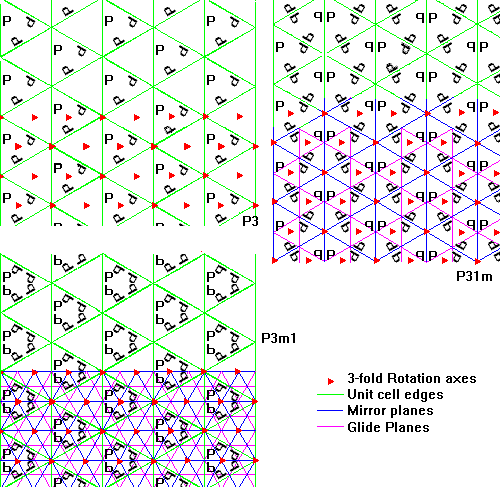Examples of each symmetry are shown below.### Hexagonal Lattices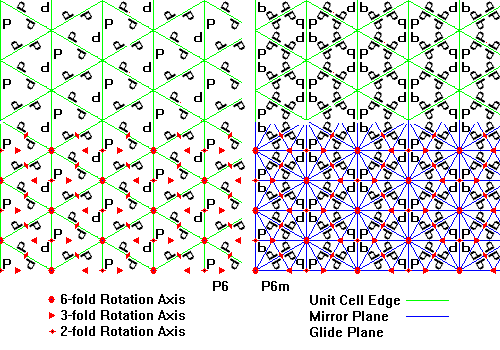Examples of each symmetry are shown below.## Symmetry Elements of the Two-Dimensional Space GroupsCreated 17 Sep 1997, Last Updated 10 June 2020